previous <<==>> next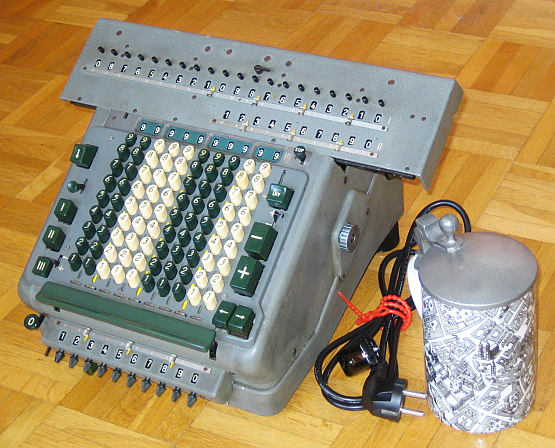``` TECHNICAL DATA of the Full-Motorized Electro-Mechanical Calculator ********************************************************************** MADAS Model 20 A Serial Number: 74921 Dimensions: (ca.) Width = 14_1/4" / 36 cm Depth = 12_1/4" / 31 cm Height = 8_3/4" / 22 cm Weight: (ca.) 35 lbs / 16 kg Power Supply: 220 V AC/DC ; 0.3 A (* Motor ~360 Counts/Min ) Mechanics: 10*9 Full-Keyboard StepDrum / Staffelwalze Functions: Add, Subtract, Multiply, Divide Full-motorized Arithmetic! Registers: Arithmetic = 20 Decimals Counter = 10 Decimals Input = 10 Decimals Multiplicator = 10 Decimals Manufacturer: HANS W. EGLI Fabrikation von Rechenmaschinen ( O. Steiger, E.Jahnz, H.W.Egli ) Zurich / Switzerland 1950 H O W T O U S E the MADAS 20 A *************************************** (*) Motor Speed Adjustment after Replacement ```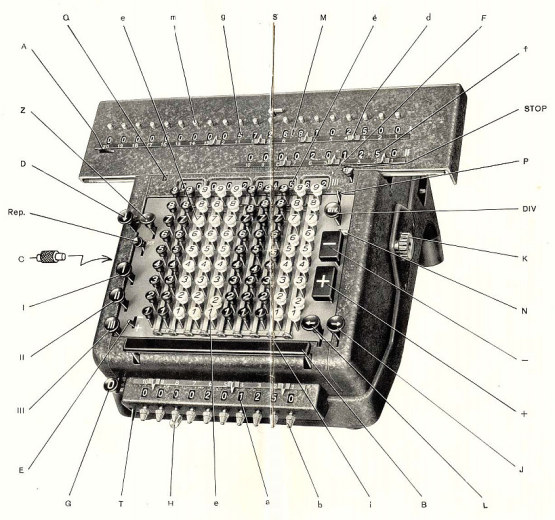```Diagram shows a similiar MADAS-Version. Refer to GERMAN TEXT down ... T H E B A S I C S : ===================== (A) Registers: --------------- (I) ARITHMETIC Unit on top of the carriage. It can be pre-set by knobs on top - and deleted by key [I]. (II) COUNTER. The +/- Lever on the right determines the counting direction UP (= default) or DOWN. Deleted by key [II]. (III) DISPLAY of the INPUT KEYS pressed. Deleted by key [III]. ( O ) MULTIPLIER-DELETE. The MULTIPLIER can be pre-set by knobs. (B) Carriage: -------------- Keys [->] / [<-] moves the carriage left or right by motor power. The far left is the START-POSITION 1. ADDITION & SUBTRACTION: ======================= Example: 123 + 45 - 6 = 162 Clear input, counter and arithmetic units. Carriage in position 1. ADD: Enter the 1st number (123) in the far right of the input unit. Press [+] key to transfer the number into the arithmetic unit. The counting unit displays the figure 1. Enter the second number (45). Press the [+] key to add the number. The arithmetic unit displays the intermediate sum (168) and the counting unit displays figure 2. SUBTRACT: Enter the 3rd number (6). Press the [-] key. The arithmetic unit displays result (162) and the counting unit is decreased by 1. REMARK 1: The ROUND Key ( over the DELETE-Keys ) is the REPEAT. It can be locked by pushing forward; released backward. The INPUT will NOT be cleared automatically. REMARK 2: NEGATIVE RESULTS are displayed in the arithmetic unit as the COMPLEMENT of the next higher 10, 100, 1000, ... Example: -12 = 99...9988 MULTIPLICATION: =============== Example: 123 x 45 = 5535 Clear input, counter and arithmetic units. Carriage in position 1. Enter the multiplicand (123) in the far right of the input unit. Press the BAR under the input unit to transfer it to the MULTIPLIER REGISTER. The input will be deleted. Enter the multiplicator (45) in the far right of the input unit and press the BAR again. The multi- plication will be automatic calculated and the carriage returns in position 1. The result is displayed in register (I) and the multi- plicand (123) is displayed in register (II) ... R E M A R K : Using MULTIPLIER-PRESET-KNOBS can speed-up the task. Example: 123 x 45 x 6 = 33210 ( continued ) To multiply a 3rd factor, press TRANSFER KEY [!] ( over clear keys ) to transfer former result in the MULTIPLIER REGISTER. Enter the 3rd factor (6), press the BAR again. The result is shown in register (I) and the former, intermediate result is shown in register (II). DIVISION: ========= Example: 22 : 7 = 3.142857142 Remainder 6 Division requires 3 steps: (a) Set the Dividend into Arithmetic Unit: -------------------------------------- For the maximum number of decimals, shift the arithmetic unit to the far right position. Enter the dividend (22) in the far left columns by PRE-SETing the arithmetic unit (I). (b) Set the Divisor in the Keyboard: -------------------------------- Enter the divisor (7) below the dividend (22). (c) Divide: ------- Press the [DIV] key and the machine will calculate all decimals (and moves the carriage) automatically. The result (3.142857142) is in the counter unit, and the remainder (6) in the arithmetic. Have a look at "Calculating Trickies" ... I N G E R M A N ...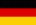====================================================================== Bemerkung: Model 20A hat KEINE "DividendenUebertragungsTaste" (Z) !!!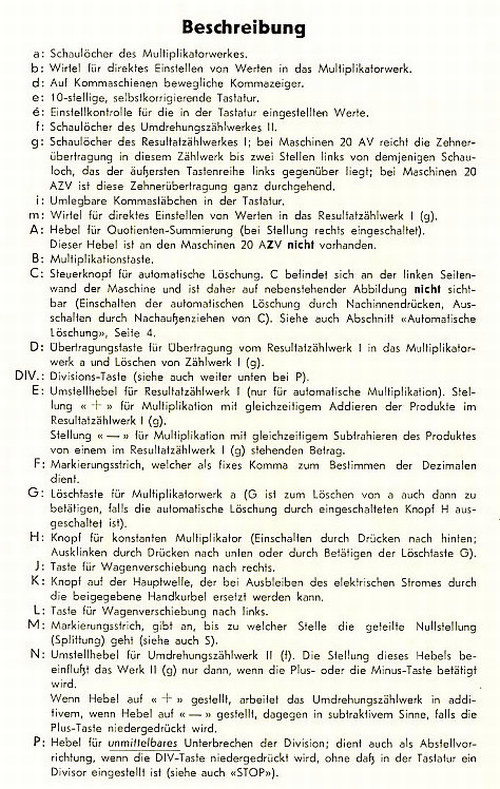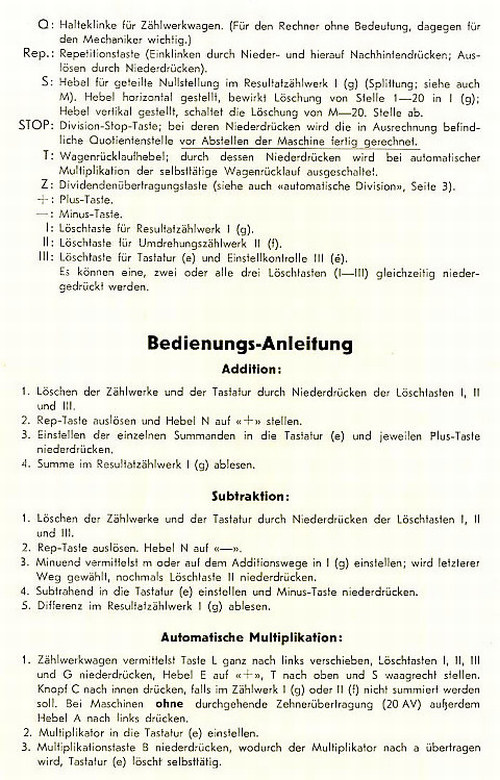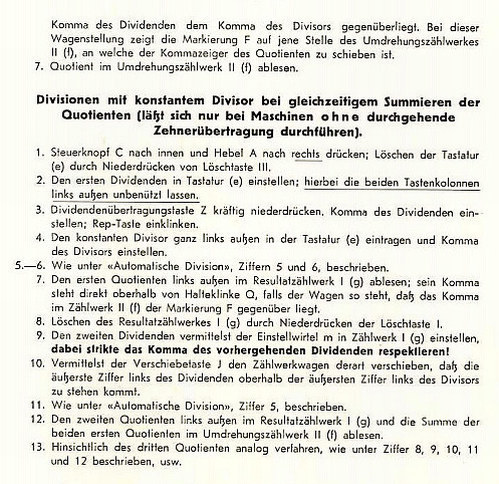R E M A R K S : PS.: The BeerStein shows "Old Munich" ---------------------------------------------------------------------- http://www.johnwolff.id.au/calculators/Tech/MadasATG/Intro.htm ... shows excellent DETAIL-PICTURES of the INNER MADAS impressum: ********************************************************************** © C.HAMANN http://public.BHT-Berlin.de/hamann 01/29/15 ```